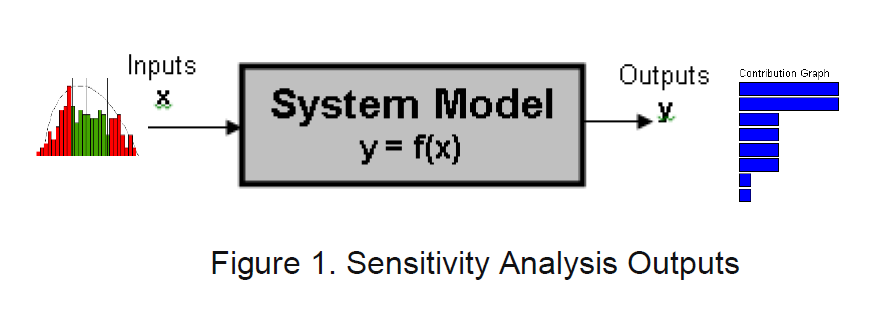<img alt="" src="https://secure.smart-enterprise-365.com/779147.png" style="display:none;">

# Sensitivity Analysis in Manufacturing Industries Whitepaper

## Sensitivity Analysis inManufacturing Industries

Sensitivity analysis is used to quantify the effects of the inputs on the outputs in a real system . Typically, the inputs can spread over a range. We would like to know how much output is affected by each input, as shown in the contributing graph in Figure 1.Sensitivity Analysis is different from Monte Carlo Simulation

Monte Carlo Simulation is trying to “replicate” the real system in two aspects: (M1) build a model to simulate a real world system; and (M2) use random-distribution-based inputs to simulate the real inputs.

Sensitivity Analysis is typically performed by using controlled inputs to calculate the results for one output. The most popular sensitivity analysis method is called “Univariate Sensitivity Analysis”. For Univariate Sensitivity Analysis, it is performed by varying one input at a time while holding other inputs at nominal values . Once the impacts from all inputs to one output are calculated, the output standard deviation can be estimated with the root-square-sum method .

Contents:

• Executive Summary
• Sensitivity Analysis is different from Monte Carlo Simulation
• Steps in Univariate Sensitivity Analysis
• Sensitivity Analysis for Manufacturing
• Contributor Analysis in 3DCS
• GeoFactor® Equation-Based Analysis in 3DCS
• Conclusion

Clean & Elegant
Fully Responsive